Part 1. Overview of Metamorphism and Tectonics
Part 2. Introduction to Metamorphism
Part 3. Physical Processes of Metamorphism
Part 4. Introductory Phase Equilibria and Thermodynamics
Part 5. Ultramafic Rocks
Part 6. Mafic Rocks
Part 7. Pelitic Rocks
Part 8. Diffusion
Part 9. Thermobarometry
Part 10. Kinetics
Part 11. Interaction Between Metamorphism and Deformation
Part 12. Metamorphism and Geochronology
Part 13. Metamorphism and Tectonics I
Part 14. Metamorphism and Tectonics II
Thermodynamics Notes

## Part 9. Thermobarometry

Read pages 53-70 of Vernon and Clarke or Chapter 16 of I&M Petrology by Best or Chapter 27 of Igneous and Metamorphic Petrology by John Winter or Chapter 19 of Igneous and Metamorphic Petrology by Philpotts

### Pressure-Temperature Paths

One of the important goals of metamorphic petrologists is to reconstruct the P-T paths experienced by rocks. In general, this goal is attained by textural analysis combined with thermobarometry.peak temperature: the maximum T experienced by a rock
peak pressure: the maximum P experienced by a rock
metamorphic field gradient: a spatially varying sequence of peak pressures and temperatures.

Qualitative thermobarometry can be done simply by inspection of rocks and minerals in the field and in thin section, based on reaction textures and mineral assemblages. These garnets show thin rims of plagioclase, likely related to decompression:

### Quantitative Thermobarometry

More sophisticated, quantitative determination of metamorphic pressures and temperatures involves four steps:

1. careful assessment of reaction textures
2. identification of zoning
3. measurement of mineral compositions
4. application of thermodynamics.

• Mineral compositions can be measured with an electron microprobe to high accuracy; they can be measured to lower detection limits with an ICP mass spectrometer.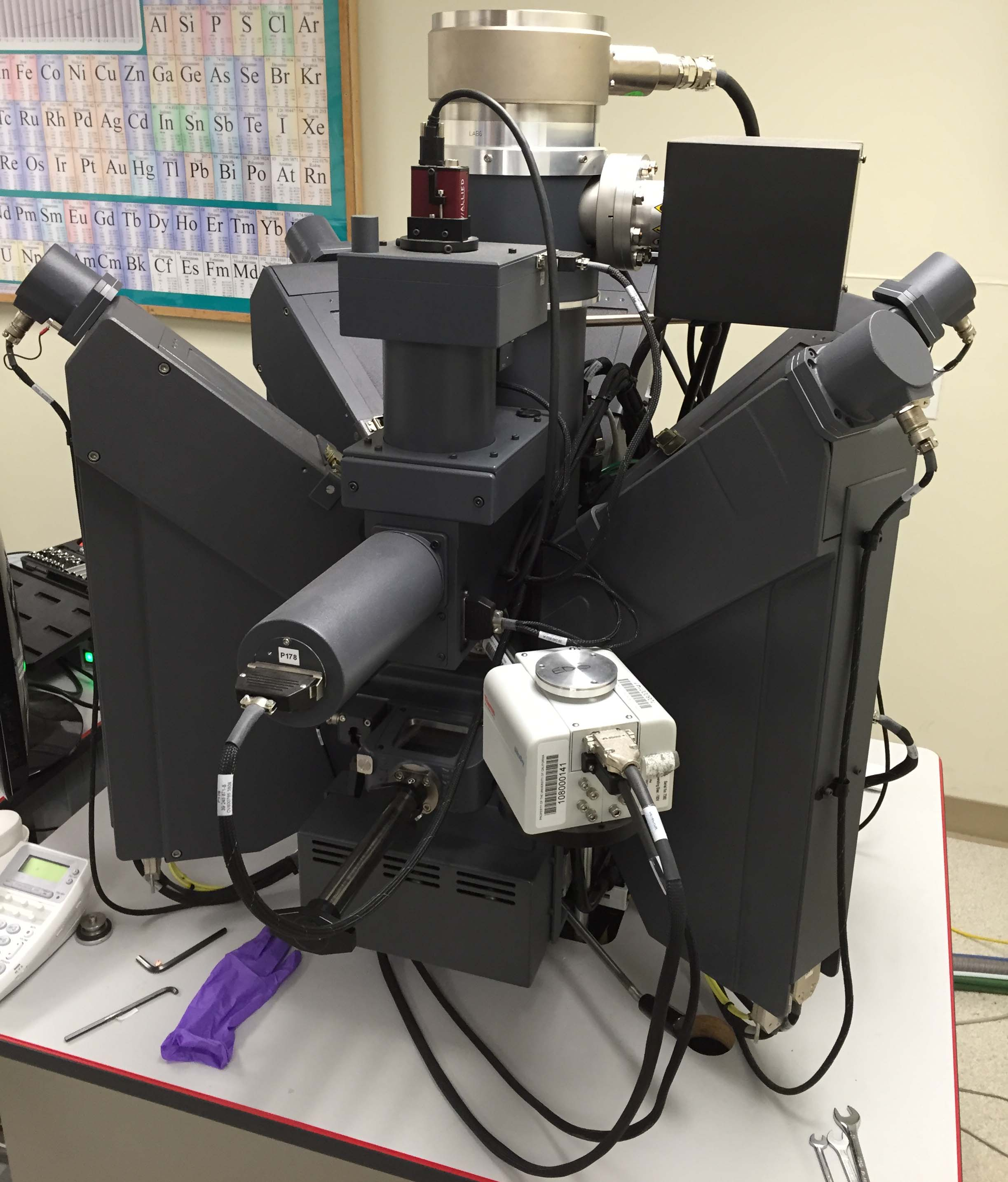1. Assessment of reaction textures. The purpose of this step is to identify which minerals are early, which are late, and which are part of a stable assemblage. Early minerals are likely to be inclusions or broken, late minerals may be in cracks or strain shadows, and minerals that are in textural equilibrium should not be separated by reaction zones. Back-scattered electron imaging is often a convenient and powerful way to study textures:This back-scattered electron image shows zoning in titanite from a Norwegian gneiss: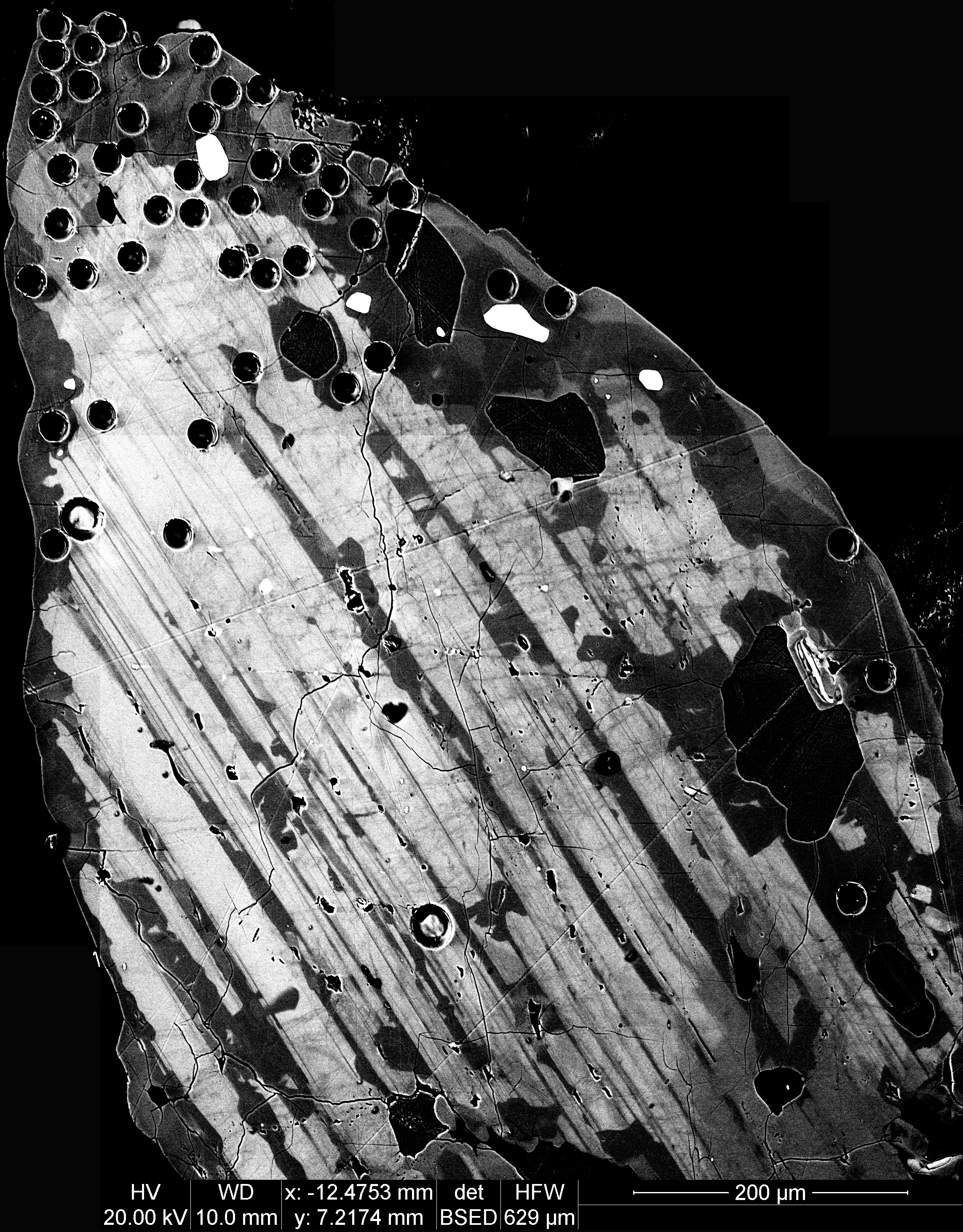2. Identification of zoning. Compositional zoning in minerals can be done by tuning the electron microprobe spectrometers--or focusing the ICP mass spectrometer detectors--to specific elements and then making element maps: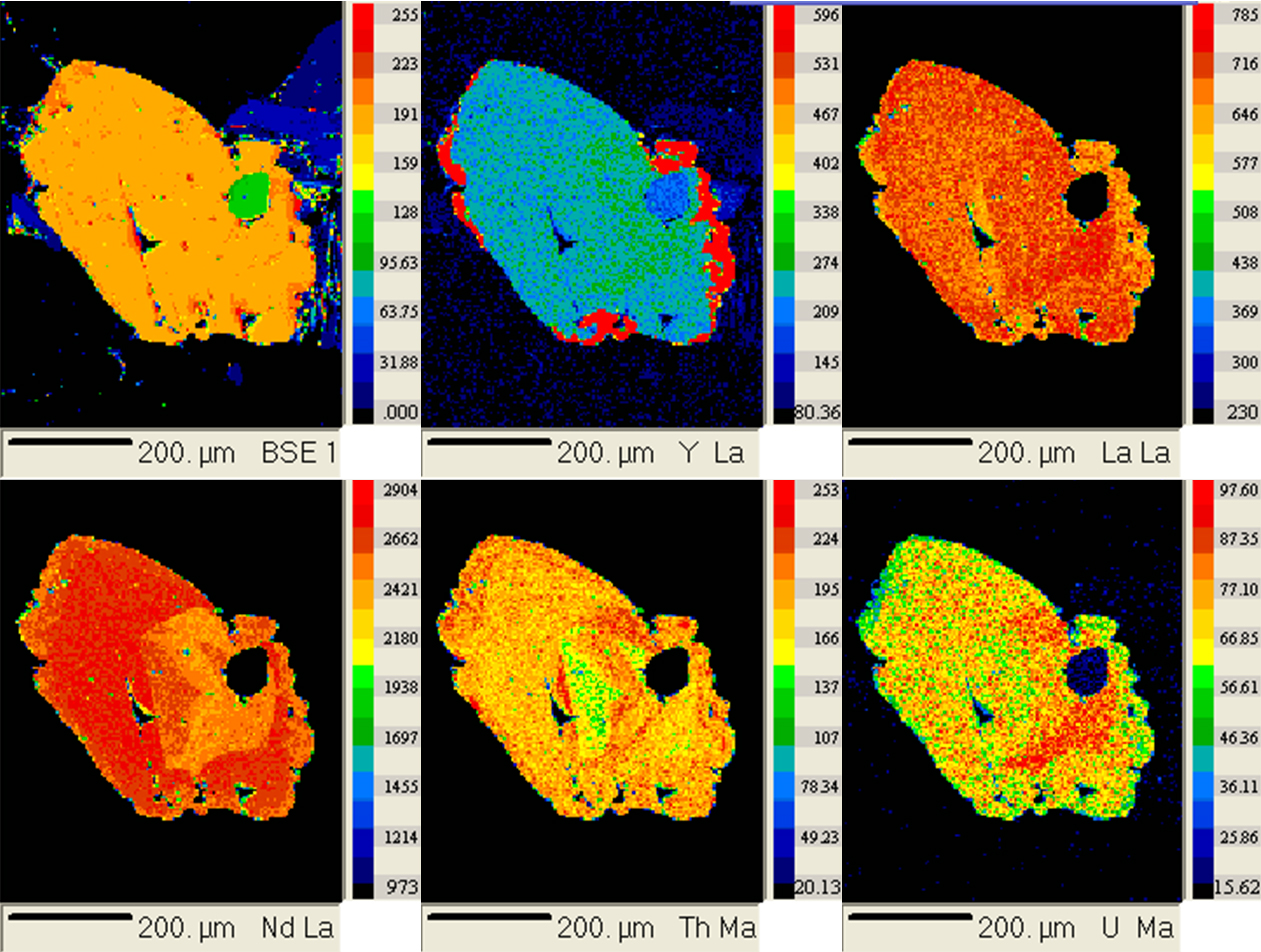An electron microprobe works by measuring element-characteristic x-rays emitted by a sample being bombarded with electrons on a 2-micron spot:Zoning can be quantified over an entire grain with good coverage of spot analyses:Such zoning can quantified using line scanning:3. Application of thermodynamics. Experiments have been used to determine the PT positions of many different kinds of endmember reactions.
For example, experiments have shown that the distribution of Fe and Mg between garnet and biotite is a function of inverse temperature: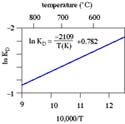thermometry: The assessment of metamorphic temperatures.
Quantitative thermometry typically is based on the exchange of Fe and Mg between pairs of minerals. For example, the exchange of Fe and Mg between garnet and biotite can be written as a reaction among the Fe and Mg endmembers of these minerals:

• Fe3Al2Si3O12 + KMg3AlSi3O10(OH)2 = Mg3Al2Si3O12 + KFe3AlSi3O10(OH)2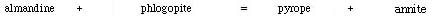barometry: The assessment of metamorphic pressures.
Qualitative barometry is based on mineral assemblages-such as the coexistence of kyanite + staurolite.
Quantitative barometry typically is based on net transfer reactions
For example, garnet - aluminumsilicate - silica - plagioclase (GASP)

• 3CaAl2Si2O8 = Ca3Al2Si3O12 + 2Al2SiO5 + SiO2
• 3 anorthite = grossular + 2 kyanite + quartz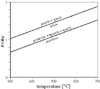### Examples of P-T Determinations

Let's look at some examples of quantitative determinations of metamorphic pressures and temperatures.
Pressures and temperatures of equilibration in lower crustal xenoliths from Tibet were constrained using compositions of garnet, pyroxene, and plagioclase.

The Fe-Mg garnet-cpx reaction is
• almandine + diopside = pyrope + ferrosilite.
One Al-garnet-opx reaction is
• CaMgSi2O6 diopside + CaAl2SiO6 'cats' = Ca2MgAl2Si3O12 garnet or
• CaFeSi2O6 hedenbergite + CaAl2SiO6 'cats' = Ca2FeAl2Si3O12 garnet
The GOPS reaction is
• grossular + 2 pyrope + 3 quartz = 3 enstatite + 3 anorthite or
• grossular + 2 almandine + 3 quartz = 3 ferrosilite + 3 anorthite.

## A Detailed Example

Here is an example, using a rock from the Pamir of Tajikistan, of the result of an integrated approach to thermobarometry that uses phase-stability fields, exchange thermometry, net-transfer reactions, and garnet zoning to determine a P-T history.## Quantitative Geothermometry and Geobarometry

Quantitative thermometry and barometry are based on thermodynamics.

### Clapeyron Relation

There is a useful relation between the slope of a reaction in PT space (i.e., dP/dT) and the entropy and volume changes of the reaction that follows from
•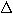rG =VrdP -SrdT
At equilibriumG = 0, such that
•rVdP =rSdT
or
•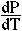=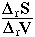So, the P-T slope of a reaction is equal to the ratio of the entropy change to the volume change. Alternatively, along the equilibrium curve, the changes in pressure times the volume change are equal to changes in temperature times the entropy change. This is the Clapeyron Equation.

Popular thermometers include garnet-biotite (GARB), garnet-clinopyroxene, garnet-hornblende, and clinopyroxene-orthopyroxene; all of these are based on the exchange of Fe and Mg, and are excellent thermometers becauserV is small, such that

•=is large (i.e., the reactions have steep slopes and are little influenced by pressure). In contrast, as we will see, reactions with gentle slopes are the best barometers.

### Calculating the PT Position of a Reaction

BecauserG = 0 at equilibrium, we can write the following approximation (ignoring fluids and variable heat capacities):
• 0 =rH1,Tref - TrS1,Tref +rVsP
and thus we can calculate the pressure of a reaction at different temperatures by
• P =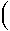rH1,Tref - TrS1,Tref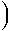/ -rVs
and we can calculate the temperature of a reaction at different pressures by
• T = Tref +rH1,Tref +rVsP/rS1,Tref
Let's do this for the albite = jadeite + quartz reaction at T = 400 K and T = 1000 K:
• P = (15,860 - 5147 * 1000) / -1.7342 = 20.6 kbar
Assuming that dP/dT is constant (incorrect in detail), the reaction looks like this

This is fine and dandy for determining P and T based on the appearance and disappearance of minerals, but how do we determine P and T based on changes in mineral composition?

### Equilibrium Constant

For the reaction
• aA + bB = cC + dD
we write the equilibrium constant:
• K = (aCc aDd/aAa aBb)
Moreover,
•rG° = -RT ln K
or
• ln K = -rG°/RT
At equilibrium, whererG°= 0, ln K = 0 and K = 1. This enables us to write a very important relationship:
• ln K = - (rH1,Tref - TrSTref +rVsP)/ RT

Let's see what K looks like for jadeite + quartz = albite at 800 K and 20 kbar:

• = -(15,860 - 800 * 51.47 + 1.7342 * 20,000)/(8.314*800) = -1.4
If we do this for all of PT space, we can contour PT space in terms of lnK:

### Solid Solutions

Almost no phases are pure, but typically are mixtures of components. For example, olivine varies from pure forsterite Mg2SiO4 to pure fayalite Fe2SiO4, and can have any composition in between--it is a solid solution. We need a way to calculate the thermodynamic properties of such solutions.
As a measure of convenience, we use mole fraction to describe the compositions of phases that are solid solutions. For example, a mix of 1 part forsterite and 3 parts fayalite yields an olivine with 25 mol% forsterite and 75 mol% fayalite, which can be written as (Mg0.25Fe0.75)2SiO4 or fo25fa75, etc. Mole fractions are denoted as Xi.

### Activity Models (Activity-Composition Relations)

Garnets are solid solutions of
component abbrev. formula
pyrope prp Mg3Al2Si3O12
almandine alm Fe3Al2Si3O12
grossular grs Ca3Al2Si3O12
spessartine sps Mn3Al2Si3O12

The simplest type of useful activity model is the ionic model, wherein we assume that mixing occurs on crystallographic sites. For a Mg-Fe-Ca-Mn garnet with mixing on one site, which we can idealize as (A,B,C,D)Al2Si3O12, the activities are
• aprp =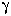Mg3XMg3
• aalm =Fe3XFe3
• agrs =Ca3XCa3
• asps =Mn3XMn3

In general, for ideal mixing in a mineral with a single crystallographic site that can containions,
• ai = Xjwhere a, the activity of component i, is the mole fraction of element j raised to thepower.

### Exchange Reactions

Many thermometers are based on exchange reactions, which are reactions that exchange elements but preserve reactant and product phases. For example:
 Fe3Al2Si3O12 + KMg3AlSi3O10(OH)2 = Mg3Al2Si3O12 + KFe3AlSi3O10(OH)2 almandine + phlogopite = pyrope + annite

We can reduce this reaction to a simple exchange vector:
• (FeMg)gar+1 = (FeMg)bio-1
 Let's write the equilibrium constant for the GARB exchange reaction K = (aprpaann)/(aalmaphl) thusrG = -RT ln (aprpaann)/(aalmaphl) This equation implies that the activities of the Fe and Mg components of biotite and garnet are a function of Gibbs free energy change and thus are functions of pressure and temperature. If we assume ideal behavior (= 1) in garnet and biotite and assume that there is mixing on only 1 site aalm = Xalm3 = [Fe/(Fe + Mg + Ca + Mn)]3 aprp = Xprp3 = [Mg/(Fe + Mg)]3 aann = Xann3 = [Fe/(Fe + Mg)]3 aphl = Xphl3 = [Mg/(Fe + Mg)]3 Thus the equilibrium constant is K = (XMggar XFebio)/(XFegar XMgbio)
• or K = (Xprp3 Xann3)/(Xalm3 Xphl3)
Long before most of you were playground bullies (1978) a couple of deities named John Ferry and Frank Spear measured experimentally the distribution of Fe and Mg between biotite and garnet at 2 kbar and found the following relationship:If you compare their empirical equation ln K = -2109 / T + 0.782 this immediately reminds you of ln K = - (rG° / RT) = -(rH / RT) - (PrV / RT) + (rS / R) Molar volume measurements show that for this exchange reactionrV = 0.238 J/bar, thusrH = 52.11 kJ/mol
The full equation is then

• 52,110 - 19.51*T(K) + 0.238*P(bar) + RT ln K = 0
To plot the K lines in PT space

### Net-Transfer Reactions

Net-transfer reactions are those that cause phases to appear or disappear. Geobarometers are often based on net-transfer reactions becauserV is large and relatively insensitive to temperature. A popular one is GASP:
 3CaAl2Si2O8 = Ca3Al2Si3O12 + 2Al2SiO5 + SiO2 anorthite = grossular + kyanite + quartz

which describes the high-pressure breakdown of anorthite.For this reactionrG = -RT ln [(aqtzaky2agrs) / aan3] = -RT ln agrs / aan3 (the activities of quartz and kyanite = 1 because they are pure phases). A best fit through the experimental data for this reaction by Andrea Koziol and Bob Newton yields P(bar) = 22.80 T(K) - 7317 forrV = -6.608 J/bar. Again, if we use ln K = -(rH / RT) - (PrV / RT) + (rS / R) and set ln K = 0 to calculate values at equilibrium, we can rewrite the above as (PrV / R) = -(rH / R) + (TrS / R) or P = TrS /rV -rH /rV if TrS /rV = 22.8 thenrS = -150.66 J/mol K ifrH /rV = 7317 thenrH = -48.357 kJ/mol
So, we can write the whole shmear as

• 0 = -48,357 + 150.66 T(K) -6.608 P (bar) + RT ln K
Contours of ln K on a PT diagram for GASP look like this:

### A Complete Example

Let's suppose you analyze a group of minerals that are in equilibrium and find the following compositions:
• garnet: Ca0.42Mg0.51Fe2.04Mn0.03Al2Si3O12
• biotite: KMg1.62Fe1.38AlSi3O10(OH)2
• plagioclase:Na0.64Ca0.36Al1.36Si2.64O8
• kyanite
• quartz
Let's determine the equilibrium P and T.
1. Determine mole fractions
• Xgrs = 0.14
• Xprp = 0.17
• Xalm = 0.68
• Xann = 0.46
• Xphl = 0.54
• Xan = 0.36
2. Determine activities, assuming ideal behavior
• agrs = 0.143 = 0.0027
• aprp = 0.173 = 0.0049
• aalm = 0.683 = 0.31
• aann = 0.463 = 0.097
• aphl = 0.543 = 0.16
• aan = 0.36
3. Calculate equilibrium constants:
• KGASP = agrs / aan3 = 0.0025 / 0.363 = 0.054
• KGARB = (aprpaann) / (aalmaphl) = (0.0049 * 0.097) / (0.31 * 0.16) = 0.0096
4. Calculate P and T:
• GASP: P (bar) = ( -48,357 + 150.66 * T(K) + RT ln K ) / 6.608
• GARB: P (bar) = ( 52,110 - 19.51 * T(K) + RT ln K ) / -0.238

Choose T = 873 K:

• GASP P = 1.7 kbar
• GARB P = -7.7 kbar

Choose T = 1073 K:

• GASP P = 13.2 kbar
• GARB P = 40.6 kbar

These two lines intersect at ~955K and 10.9 kbar.Part 1. Overview of Metamorphism and Tectonics
Part 2. Introduction to Metamorphism
Part 3. Physical Processes of Metamorphism
Part 4. Introductory Phase Equilibria and Thermodynamics
Part 5. Ultramafic Rocks
Part 6. Mafic Rocks
Part 7. Pelitic Rocks
Part 8. Diffusion
Part 9. Thermobarometry
Part 10. Kinetics
Part 11. Interaction Between Metamorphism and Deformation
Part 12. Metamorphism and Geochronology
Part 13. Metamorphism and Tectonics I
Part 14. Metamorphism and Tectonics II
Thermodynamics Notes